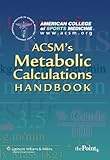Home FAQ Random quotes Contact

# ACSM's Metabolic Calculations HandbookISBN13: 9780781742382
ISBN: 0781742382
Binding: Paperback
List Price: \$32.99
Publisher: Lippincott Williams & Wilkins
Published Date:
Edition 1
Pages: 128
Average Goodreads rating: 3.57/5 (6 ratings)

This handbook provides a step-by-step approach to using metabolic equations, from basic math principles to applying the equations to an exercise plan. Chapters focus separately on each equation, provide an easy-to-follow process of solving, and demonstrate the varied uses of the equation in clinical as well as fitness settings.

Each chapter includes a set of problems that focus on real-world applications of the equation. Step-by-step problem solution explanations are provided at the end of each chapter. A comprehensive exam at the end of the book tests the reader's skill in using the equations.